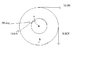# Torque and vector product

• aaronb

## Homework Statement

find the net torque on the wheel about the axle through O, taking a = 7.00cm and b = 20.00cm. Assume the positive direction is counterclockwise

## Homework Equations

torque = force x distance
torque = force x (radius x sin(phi))

## The Attempt at a Solution

10.0 x .2m = -2 (because it's moving clockwise)
9.00 x .2m = -1.8
.07 x 12 x sin(30) = .42

net torque = -3.38 (this is off by 10%-100%)

#### Attachments

•physicshw10and11prob7.jpg
12 KB · Views: 382
I don't understand what the 30 degree angle represents.

Me niether, do you think it was put there to confuse?

aaronb said:
Me niether, do you think it was put there to confuse?
Beats me. It looks like the 12 N force is tangential to the circle of radius a. Is that the case?

It is there to confuse. The net torque turned out to be -2.96 so the 12N force is tangential. Thanks for bringing that up to attention